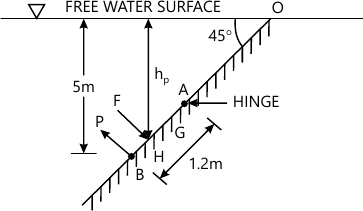MORE IN Mechanics of Fluids
VTU Civil Engineering (Semester 3)
Mechanics of Fluids
December 2014
Total marks: --
Total time: --
INSTRUCTIONS
(1) Assume appropriate data and state your reasons
(2) Marks are given to the right of every question
(3) Draw neat diagrams wherever necessary

1 (a) State and prove Newton's law of viscosity.
4 M
1 (b) The space between two square flat parallel plates is filled with oil. Each side of the plate is 60cm. The thickness of the oil film is 12.5m. The upper plate, which moves at 2.5 metre per sec requires a force of 98.1 N to maintain the speed. Determine i) the dynamic viscosity of the oil in poise and ii) the kinematic viscosity of the oil in strokes if the specific gravity of oil is 0.95.
6 M
1 (c) Define capillarity and derive an expression for capillary rise.
5 M
1 (d) Calculate the capillary effect in mm in a glass tube of 4mm diameter, when immersed in, i) Water and ii) mercury. The temperature of the liquid is 20°C and the values of the surface tension of water and mercury at 20°C in contact with air are 0.073575 N/m and 0.51 N/m respectively. The angle of contact for water is zero and that for mercury is 130°. Take density of water at 20°C as equal to 998 kg/m3.
5 M

2 (a) Derive Pascal's law for the intensity of pressure at a point in a static fluid.
6 M
2 (b) Differentiate between i) Absolute pressure and Gauge pressure ii) Simple manometer and differential manometer.
6 M
2 (c) A differential manometer is connected at the two points A and B of two pipes. The centre of pipe A is 3m above centre of pipe B. Pipe A contains liquid of sp.gr. 1.5 while pipe B contains a liquid of sp.gr. 0.9. The manometric liquid mercury is 5m below the centre of pipe A. The pressure at A and B are 1 Kgf/cm2 and 1.8 Kgf/cm2 respectively. Find the difference in mercury level in the differential manometer.
8 M

3 (a) Derive an expression for total pressure and centre of pressure for a vertical plane surface submerged in liquid,
8 M
3 (b) Determine the total pressure and centre of pressure on an isosceles triangular plate of base 4m and altitude 4m when it is immersed vertically in an oil of sp.gr. 0.9. The base of the plate coincides with the free surface of oil.
6 M
3 (c) An inclined rectangular sluice gate AB, 1.2 m by 5 m size as shown in Fig. Q3(c) is installed to control the discharge of water. The end A is hinged. Determine the force normal to the gate applied at B to open it.6 M

4 (a) Define equation of continuity. Derive an expression for continuity equation for a three-dimensional flow.
8 M
4 (b) A Fluid flow field is given by,
V=x2yi+y2zj-(2xyz+yz2)k
Prove that it is a case of possible steady incompressible fluid flow. Calculate the velocity and acceleration at the point (2,1,3).
12 M

5 (a) Derive Euler's equation of motion.
6 M
5 (b) The water is flowing through a pipe having diameters 20 cm and 10 cm at sections 1 and 2 respectively. The rate of flow through pipe is 35 litres/s. The section 1 is 6 m above datum and section 2 is 4 above datum. If the pressure at section 1 is 39.24 N/cm2, find the intensity of pressure at section 2.
7 M
5 (c) The inlet and throat diameters of a horizontal venturimeter are 30cm and 10cm respectively. The liquid flowing through the meter is water. The pressure intensity at inlet is 13.734 N/cm2 while the vacuum pressure head at the throat is 37 cm of mercury. Find the rate of flow. Assume that 4% of the differential head is lost between the inlet and throat. Find also the value of Cd for the venturimeter.
7 M

6 (a) An oil of sp.gr. 0.9 and viscosity 0.06 poise is flowing through a pipe of diameter 200 mm at the rate of 60 litres/s. Find the head lost due to friction for a 500 m length of pipe. Find the power required to maintain this flow.
6 M
6 (b) At a sudden enlargement of water main from 240 mm to 480 mm diameter, the hydraulic gradient rises by 10 mm. Estimate the rate of flow.
8 M
6 (c) Water is flowing through a horizontal pipe of diameter 200 mm at a velocity of 3 m/s. A circular solid plate of diameter 150mm is placed in the pipe to obstruct the flow. Find the loss of head due to obstruction in the pipe if CC = 0.62.
6 M

7 (a) Explain any five methods of measuring water depth with the help of neat sketch.
10 M
7 (b) Describe the area velocity method to measure discharge through a stream section.
5 M
7 (c) A pitot-static tube having a coefficient of 0.98 is used to measure the velocity of water in a pipe. The stagnation pressure recorded is 3 m and the static pressure 2m. Determine the velocity.
5 M

8 (a) Explain the procedure to measure discharge using
i) Triangular notch
ii) Cipolletti notch
Orificemeter.
12 M
8 (b) A broad-crested weir of 50 m length, has 50 cm height of water above its crest. Find the maximum discharge. Take Cd=0.60. Neglect velocity of approach Also, if the velocity of approach is to be taken into consideration, find maximum discharge when the channel has a cross sectional area of 50 m2 on the upstream side.
8 M

More question papers from Mechanics of Fluids